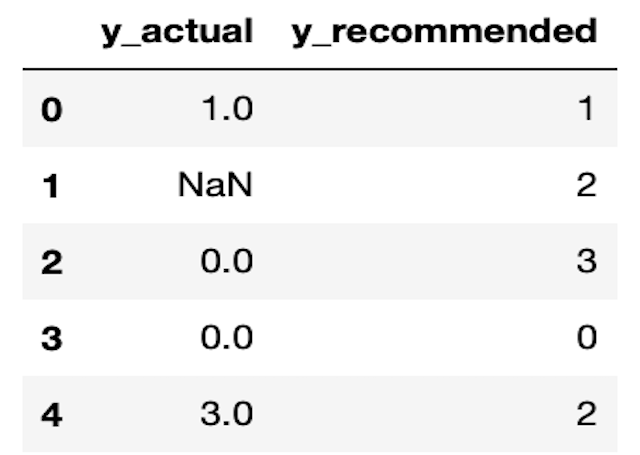# Classification

This category groups articles on the subject of classification. Each post focuses on either a specific classification algorithm, or a tool used when tackling a classification problem. The emphasis here is on understanding these models and techniques at a technical level. Here you will learn to build classification models in Python from scratch.## Can Decision Trees Handle Categorical Features?

Can Decision Trees Handle Categorical Features? Yes, Decision Trees handle categorical features naturally. Often these features are treated by first one-hot-encoding (OHE) in a preprocessing step. However, it is straightforward to extend the CART algorithm to make use of categorical features without such preprocessing. In this post, I will implement classification and regression Decision Trees capable …## Can Decision Trees Handle Missing Values?

Can Decision Trees Handle Missing Values? Yes, Decision Trees handle missing values naturally. It is straightforward to extend the CART algorithm to support the handling of missing values. However, attention needs to be made regarding how the algorithm is implemented in code. In this post, I will implement classification and regression Decision Trees capable of dealing …## 3 Methods to Tune Hyperparameters in Decision Trees

3 Methods to Tune Hyperparameters in Decision Trees We can tune hyperparameters in Decision Trees by comparing models trained with different parameter configurations, on the same data. An optimal model can then be selected from the various different attempts, using any relevant metrics. There are several different techniques for accomplishing this task. Three of the …## How to Measure Information Gain in Decision Trees

How to Measure Information Gain in Decision Trees For classification problems, information gain in Decision Trees is measured using the Shannon Entropy. The amount of entropy can be calculated for any given node in the tree, along with its two child nodes. The difference between the amount of entropy in the parent node, and the …## Precision@k and Recall@k Made Easy with 1 Python Example

Understanding the Adaboost Regression Algorithm For those who prefer a video presentation, you can see me work through the material in this post here: https://youtu.be/WEJcETfWwOo What are Precision@k and Recall@K ? Precision@k and Recall@k are metrics used to evaluate a recommender model. These quantities attempt to measure how effective a recommender is at providing relevant suggestions …